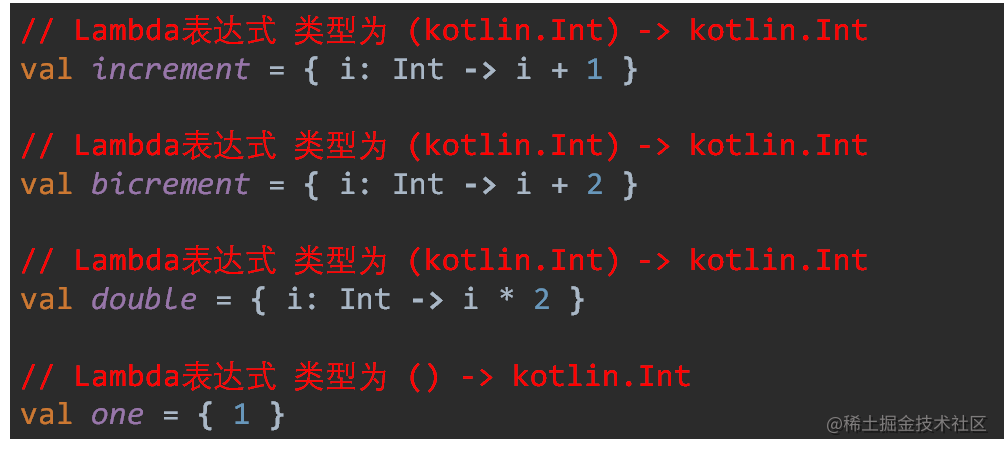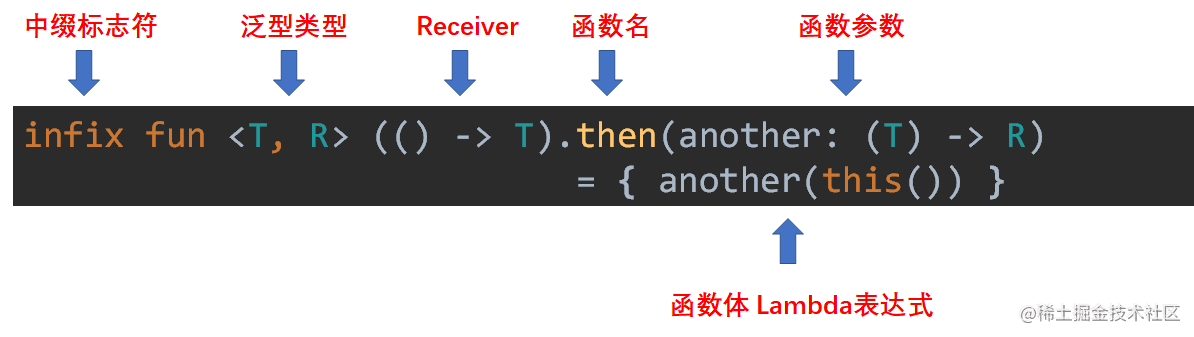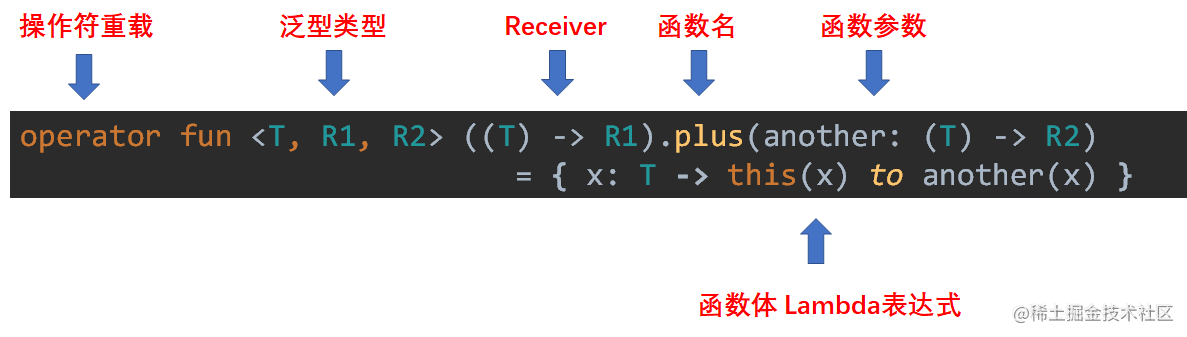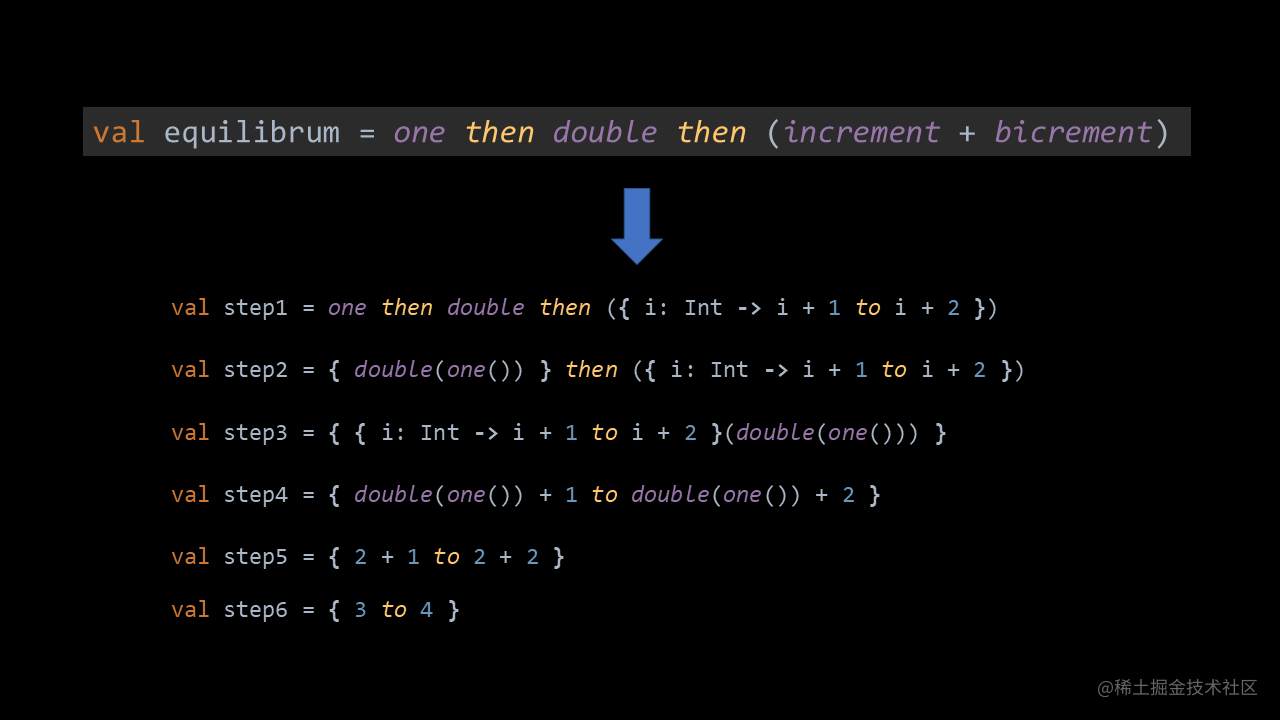# 有趣的 Kotlin 0x0A：Fun with composition

【有趣的 Kotlin 】系列记录自己对每一题的理解。

## 0x0A：Fun with composition

``````val increment = { i: Int -> i + 1 }
val bicrement = { i: Int -> i + 2 }
val double = { i: Int -> i * 2 }
val one = { 1 }
​
infix fun <T, R> (() -> T).then(another: (T) -> R) = { another(this()) }
operator fun <T, R1, R2> ((T) -> R1).plus(another: (T) -> R2) = { x: T -> this(x) to another(x) }
​
fun main(args: Array<String>) {
val equilibrum = one then double then (increment + bicrement)
print(equilibrum())
}

1. Nothing, it doesn't compile
2. 5
3. (1,2)
4. (3,4)

## 分析

### 先看看变量• increment：加 1
• bicrement：加 2
• double：加倍
• one：调用后返回数值 1

### 再看看函数

4 个变量后，紧跟 2 个函数，一个是中缀函数，一个是操作符重载函数。

• `then`：串联两个函数。先执行 `receiver`，结果作为参数传入 `another`
• `plus`：合并两个函数的结果。执行 `receiver` ，执行 `another`，并将两个结果组成 `Pair`

#### 中缀函数 then`then` 函数是一个中缀函数，同时也是一个泛型函数，持有 `T`, `R` 两种泛型类型

• receiver：`() -> T`
• 参数：`(T) -> R`
• 返回值：`() -> R`

• 必须是成员函数或扩展函数。
• 必须有唯一的函数参数。
• 参数不可为可变数量的参数 (vararg )，并且不能有默认值。
``````infix fun Int.shl(x: Int): Int { ... }
​
// calling the function using the infix notation
1 shl 2
​
// is the same as
1.shl(2)

#### 操作符重载函数 plus`plus` 是一个操作符重载函数，同时也是一个泛型函数，持有 `T``R1``R2` 三种泛型类型。

• receiver：`(T) -> R1`
• 参数：`(T) -> R2`
• 返回值：`(T) -> Pair<R1, R2>`

### 最后看主逻辑

``````fun main(args: Array<String>) {
val equilibrum = one then double then (increment + bicrement)
println(equilibrum())
}`Pair` 类型的 `toString()` 方法 `public override fun toString(): String = "(\$first, \$second)"`

## 总结

`````` val equilibrum = one then double then (increment + bicrement)

\